## Write an equation of a line that passes through (3, -1) and is perpendicular to y = 6x – 4.

Question

Write an equation of a line that passes through (3, -1) and is perpendicular to y = 6x – 4.

in progress 0
5 months 2021-09-05T12:25:59+00:00 2 Answers 0 views 0

## Answers ( )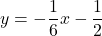Step-by-step explanation:

We want to write the equation of a line that passes through the point (3, -1) and is perpendicular to the line: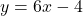Remember that the slopes of perpendicular lines are negative reciprocals of each other.

The slope of the given equation is 6.

So, the slope of our perpendicular line is the negative reciprocal of 6, which is -1/6.

Next, we are given that it passes through the point (3, -1). Since we are given a point and know the slope, we can use the point-slope form. Point-slope form is given by: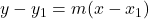Where m is the slope and (x₁, y₁) is a point.

We will substitute -1/6 for m and (3, -1) for (x₁, y₁). Hence: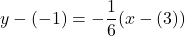Simplify: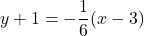Our answer is valid as it as, but we can simplify this into slope-intercept form. Distribute: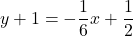And subtract one from both sides. Therefore, our equation is: